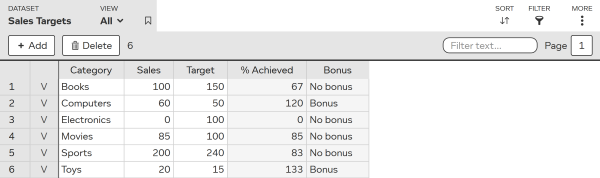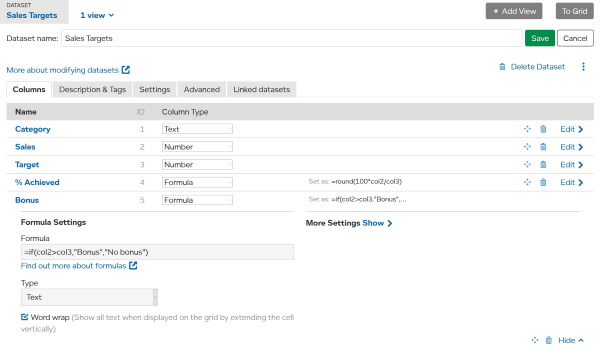## Advanced LiveDataset

Link datasets together to build online systems.

# Add Calculated Columns

## What are Calculated Columns?

A dataset column can be calculated from other columns by selecting the “Formula” column type and entering a formula. This formula will be automatically calculated for all entries in the dataset, including future entries added to the dataset.

This dataset has 2 calculated columns showing percentage of sales target achieved and if a bonus has been awarded. Calculated columns are always shown with a grey background as the values are read-only.## How to Add a Calculated Column

Modify the dataset and add a column, selecting “Formula” as the column type. Then enter a formula. The results of the formula can be shown as Text or Number.

TIP Press Edit if the Formula Settings are not shown.In this example, the two formulas used are

Column Formula
% Achieved =round(100*col2/col3)
Bonus =if(col2>col3,“Bonus”,“No bonus”)

When a formula refers to a column, use “col” and the column ID number. In the example above the ID for Sales is 2, so in the formula we use `col2`.

A formula can be as simple as

• =100
• =”Hello World”
• = col2 + col3

Formulas can contain numbers, text, and functions including

These functions are described in Using Formulas and Functions